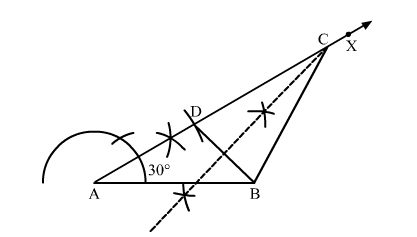# Construct a ∆ABC in which base AB = 5 cm, ∠A = 30° and AC – BC = 2.5 cm.

Question:

Construct a ∆ABC in which base AB = 5 cm, ∠= 30° and AC – BC = 2.5 cm. Justify your construction.

Solution:Steps of construction:
1. Draw a line segment AB = 5 cm.

2. Construct $\angle B A X=30^{\circ}$.

3. Set off AD = 2.5 cm.
4. Join DB.
5. Draw the right bisector of DB, meeting DB produced at C.
6. Join CB.

Thus, $\triangle A B C$ is the required triangle.

Justification:
Point C lies on the perpendicular bisector of DB.
⇒ CD = BC
Now
AD = AC − DC = AC − BC